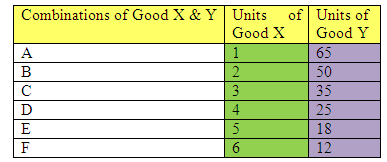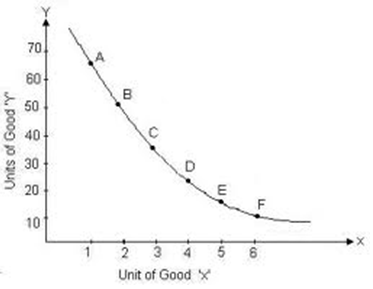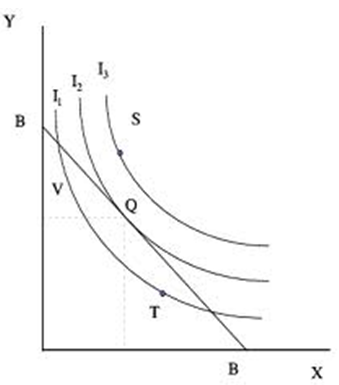# Indifference Curve, Marginal Rate of Substitution, Microeconomics Assignment Help

###The figure above represents distinct combinations of two goods i.e. X and Y. At each point on the curve i.e. A, B, C, D, E and F, the consumer does not have to make any preference for one over the other i.e. all the above presented combinations are evenly preferred and offer the equal degree of satisfaction to the buyer. Marginal Rate of Substitution With the purpose to remain on the same Indifference Curve, the customer has to give up one good for another by just same amount which continues to maintain him/her on the same level of satisfaction. In other words, the marginal rate of substitution is the rate at which the buyer is willing to replace one good for another in order to be on the same level of contentment. For instance, there are two goods X and Y and the consumer is ready to give up X for Y. So, marginal rate of substitution of x for y = Δy/Δx MRS xy = Δy/Δx Budget Line Budget line can be described as a line which represents distinct combinations of two goods that can be purchased in a definite or given income. The budget line graph is a downward sloping line whose gradient represents the ratio between the prices of two goods X and Y. When there is an increment in the income of the buyer, the capability to purchase also goes up, consequently the budget line moves parallel towards the right side. While when there is a fall in income, the budget line shifts parallel towards the left side. Consumer’s Equilibrium A consumer is said to be in equilibrium only at the point where he obtains maximum utility keeping in mind the given budget. Moreover, the point where the budget line and indifference curve are tangent to each other is acknowledged as the equilibrium point. At this particular point the consumer receives maximum satisfaction from the amalgamation of two available products. Further, it is the maximum indifference curve consumer can arrive at with respect to his/her budget constraints.# Why Us ?